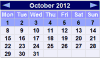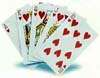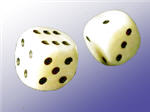# 概率：余集事件是得到正面，余集是得到反面事件是 {星期一、星期三}，余集是 {星期二、星期四、星期五、星期六、星期天}事件是 {红心}，余集是 {黑桃、梅花、方块、小丑}

## 概率

### 例子：掷骰子得到 "4"点的可能性

P(A) 的意思是 "事件 A 的概率"

P(A') 的意思是 "事件 A 的余集的概率"

P(A) + P(A') = 1### 例子：掷个 "5" 或 "6"

P(A) = 26 = 13

P(A') = 4 6 = 2 3

P(A) + P(A')  =   1 3 + 2 3   =   3 3   =  1

## 余集有什么用？### 例子：掷两个骰子。得到两个不同数字的概率是多少？

A = { (1,2)、(1,3)、(1,4)、(1,5)、(1,6)、
(2,1)、(2,3)、(2,4)……等等！}

A' = { (1,1)、(2,2)、(3,3)、(4,4)、(5,5)、(6,6) }

P(A') = 6/36 = 1/6

P(A) = 1 − P(A')
= 1 − 1/6
= 5/6